# 深度学习神经网络在力学模型参数估计中的应用研究进展Some Developments of Estimation Procedures of Mechanical Model Parameter Based on Deep Learning Neural Network

DOI: 10.12677/AIRR.2020.92012, PDF, HTML, XML, 下载: 126  浏览: 653  国家自然科学基金支持

Abstract: In order to estimate mechanical model parameters of materials, the basic scheme and algorithm of parameter inversion based on classical BP neural network are discussed. Some drawbacks and improvement methods for classical BP neural network are analyzed. Combined two examples of estimating model parameters of electric-chemical model of Li-ion cells, the basic thinking of esti-mating model parameter procedure based on deep learning neural network is introduced. How to determine hyper parameters of deep learning neural network is discussed. How to improve learning efficiency and generalization ability of deep learning neural network is developed to es-timate model parameters of materials.

1. 引言

2. 经典BP神经网络在力学模型参数估计中应用

${E}_{t}=k{P}_{a}{\left(\frac{{\sigma }_{3}}{{P}_{a}}\right)}^{n}{\left[1-\frac{{R}_{f}\left({\sigma }_{1}-{\sigma }_{3}\right)\left(1-\mathrm{sin}\varphi \right)}{2c\mathrm{cos}\varphi +2{\sigma }_{3}\mathrm{sin}\varphi }\right]}^{2}$ (1)

${B}_{t}={K}_{b}{P}_{a}{\left(\frac{{\sigma }_{3}}{{P}_{a}}\right)}^{m}$ (2)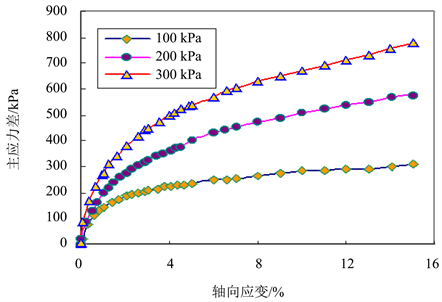Figure 1. Relationship between stress and axial strain in different confined pressureFigure 2. Relationship between radial and axial strains in different confined pressure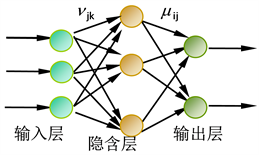Figure 3. Topology structure of BP neural network for estimating model parameters

$E\left(w\right)=\frac{1}{2}{\sum }_{p=1}^{P}\left({\sum }_{i=1}^{{N}_{o}}{\left({y}_{i}^{p}-{o}_{i}^{p}\right)}^{2}\right)$ (3)

$f\left(Sigma\right)=1/\left[1+\mathrm{exp}\left(-Sigma\right)\right]$ (4)

ReLU作用函数：深度学习神经网络除了传统的sigmoid函数之外，使用了RELU (Rectified Linear Unit)函数。当输入大于0时，该函数的输出就是输入；而当输入小于0时，该函数的输出是0。

$f\left(Sigma\right)=\mathrm{max}\left(Sigma,0\right)$ (5)

$f\left(Sigma\right)=\frac{\mathrm{exp}\left(Sigma\right)-\mathrm{exp}\left(-Sigma\right)}{\mathrm{exp}\left(Sigma\right)+\mathrm{exp}\left(-Sigma\right)}$ (6)

$f\left(Sigma\right)=Sigma$ (7)

${y}_{i}={f}_{o}\left({\sum }_{j=1}^{{N}_{h}}\left({\mu }_{ij}{z}_{j}+{\lambda }_{i}\right)\right)$ (8)

${z}_{j}={f}_{h}\left({\sum }_{k=1}^{{N}_{i}}\left({v}_{jk}{x}_{k}+{\eta }_{k}\right)\right)$ (9)

1) 基于先验信息，确定被估计力学模型参数的大致范围；随机产生训练样本集和测试集。当采用sigmoid作用函数时，输入数据需要进行归一化处理。输入数据的正规化(归一化normalization)处理，使得输入数据的值介于[−1,1]之间。

${x}_{kn}=2\left\{\left({x}_{k}-{x}_{\mathrm{min}}\right)/\left({x}_{\mathrm{max}}-{x}_{\mathrm{min}}\right)\right\}-1$ (10)

2) 基于Duncan-Chang弹性非线性本构模型，确定将要模拟的围压值，解析计算在不同力学模型参数随机组合条件下的应力–应变曲线，类似于图1图2

$\Delta {\sigma }_{d}={E}_{t}\Delta {\epsilon }_{a}$ (11)

$\Delta {\epsilon }_{r}={\mu }_{t}\Delta {\epsilon }_{a}$ (12)

3) 建立BP神经网络模型，确定神经网络的拓扑结构，随机给神经网络权值和阈值赋初始值，设定神经网络收敛准则；

4) 将训练样本集中应力–应变数据作为神经网络的输入，而力学模型中的参数作为神经网络的期望输出。基于MATLAB软件或者其他软件平台，采用优化方法训练神经网络，例如Levenberg-Marquardt优化算法，Quasi-Newton优化算法，直至神经网络收敛，记录神经网络的权值和阈值；

5) 将测试集输入到已经训练好的神经网络，检验神经网络的泛化能力和预测精度。

6) 室内进行土体三轴压缩实验，记录不同围压条件下的应力–应变曲线。将这些观测数据代入到训练好的神经网络模型，神经网络的输出就是力学模型的参数，具体的实施步骤和方法详见参考文献 。

3. 深度学习神经网络在模型参数估计中应用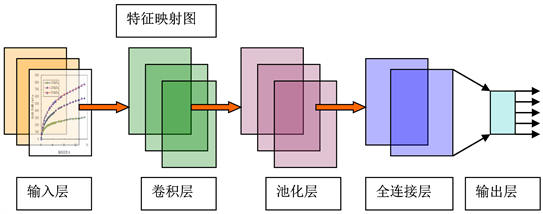Figure 4. Topology structure of convolutional neural network for estimating model parameters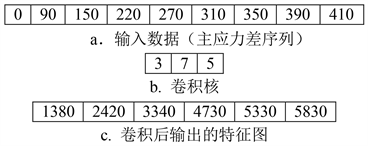Figure 5. One dimensional Convolutional operation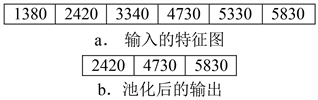Figure 6. One dimensional maximum pooling operation

1) 选定卷积神经网络的拓扑结构，确定卷积层、池化层的层数；选定核函数和池化方法；选取神经元的作用函数；

2) 选取神经网络学习的优化算法和目标函数，例如随机梯度搜索算法；确定神经网络中的超参数、收敛准则和最大迭代次数；

3) 基于解析或者数值模型，建立神经网络训练样本集和测试集；

5) 输入神经网络测试集，测试神经网络；

6) 室内进行土体三轴压缩实验，记录不同围压条件下的应力–应变曲线。

7) 将实验数据输入神经网络，估计力学模型参数。将基于神经网络反演得到力学模型参数，代入到已知的力学模型，预测岩土材料的变形曲线，验证方法的有效性。

2) 目标函数的正则化方法  ，该方法受Tikhonov正则化算法的启发，它也类似于罚函数方法，在均方误差(mean squared error)后面增加了一项

$E\left(w\right)=\frac{1}{2}{\sum }_{p=1}^{P}\left({\sum }_{i=1}^{{N}_{o}}{\left({y}_{i}^{p}-{o}_{i}^{p}\right)}^{2}\right)+\alpha {W}^{\text{T}}W$ (13)

3) 神经网络训练目标函数除了均方误差之外，还有交叉熵误差作为目标函数 ，该方法可以提高基于梯度下降法搜索最优网络权值的收敛速度。

$E\left(w\right)=-{\sum }_{p=1}^{P}{\sum }_{i=1}^{{N}_{o}}{o}_{pk}\mathrm{ln}{y}_{pi}$ (14)

4) 神经网络超参数优化方法。超参数，例如学习率(learning rate)，动量项(momentum)，隐含层神经元的个数。超参数往往需要进行多次尝试，以便找到一组合理的超参数 。神经网络超参数(hyper parameter)优化问题，与其说是一门科学方法，倒不如说是一门艺术。为了获得比较合理的一组超参数，往往采用试错的方法，经过多次试凑得到。将数据集分为训练数据、验证数据和测试数据。其中，训练数据用于神经网络学习，确定神经网络的权值和阈值，而验证数据用于评估神经网络的超参数，而测试数据用于检验神经网络的泛化能力。超参数的优化问题，可以采用贝叶斯优化方法(Bayesian optimization) 。这可能是目前限制神经网络更广泛应用的一个瓶颈。

5) 神经网络初始权值选取的问题。较差的初始权值可能会导致神经网络学习失败。同时，为了防止神经网络初始权值均一化，初始权值应该随机产生。Xavier提出了使用标准差为1/N1/2的高斯分布进行权值初始化的方法，N表示神经元的个数。

6) 抑制过拟合、提高泛化能力的问题。为了解决该问题，Srivastava提出了随机删除神经网络单元方法(Dropout方法) 。在神经网络训练过程中，随机选择隐含层的神经元，将其删除(杀死)，使其不再传递信号。但是，随机删除某些神经单元方法，删除多少个，删除原则，缺少理论依据。

7) 深度学习输入数据的预处理方法，包括归一化方法、均值减法、均一化方法、白化方法等  。

4. 结论

  Albert Tarantla. 模型参数估计的反问题理论与方法[M]. 北京: 科学出版社, 2009.  Li, S.-J. and Shao, L.-T. (2012) Inverse Procedure for Determining Model Parameter of Soils Using Real-Coded Genetic Algorithm. Journal of Central South University, 19, 1764-1770. https://doi.org/10.1007/s11771-012-1203-2  李守巨, 于申, 孙振祥. 基于神经网络的堆石料本构模型参数反演[J]. 计算机工程, 2014, 46(4): 267-271.  Lecun, Y., Bengio, Y. and Hinton, G. (2015) Deep Learning. Nature, 521, 436-444. https://doi.org/10.1038/nature14539  山下隆义. 图解深度学习[M]. 北京: 中国工信出版社, 2018.  涌井良幸. 深度学习的数学[M]. 北京: 中国工信出版社, 2019.  斋藤康毅. 深度学习入门[M]. 北京: 中国工信出版社, 2018.  Chun, H.Y. (2019) Parameter Identification of an Electrochemical Lithium-Ion Battery Model with Conventional Neural Network. IFAC Paperonline, 52, 129-134. https://doi.org/10.1016/j.ifacol.2019.08.167  Shen, S. (2019) A Deep Learning Method for Online Capacity Estimation of Lithium-Ion Batteries. Journal of Energy Storage, 25, 1-13.  Kaba, K. (2018) Estimation of Daily Global Solar Radiation Using Deep Learning Model. Energy, 162, 126-135. https://doi.org/10.1016/j.energy.2018.07.202  Radoslaw, M.C. and Aniel, K. (2019) Deep Neural Networks as Scientific Models. Trends in Cognitive Sciences, 23, 305-317.  Taesic, L., Juwon, K., Young, U. and Hyunju, L. (2019) Deep Neural Network for Estimating Low Density Lipoprotein Cholesterol. Clinica Chimica Acta, 489, 35-40. https://doi.org/10.1016/j.cca.2018.11.022  Cui, M.J. and Khodayar, M. (2019) Deep Learning Based Time-Varying Parameter Identification for System-Wide Load Modeling. IEEE Transactions on Smart Grid, 10, 6102-6114. https://doi.org/10.1109/tsg.2019.2896493  Weimer, D. and Scholz-Reiter, B. (2016) Design of Deep Convolutional Neural Network Architectures for Automated Feature Extraction in Industrial Inspection. CIRP Annals-Manufacturing Technology, 65, 417-420. https://doi.org/10.1016/j.cirp.2016.04.072  Yu, H.Y. and Khan, F. (2015) Nonlinear Gaussian Belief Network Based Fault Diagnosis for Industrial Processes. Journal of Process Control, 35, 178-200. https://doi.org/10.1016/j.jprocont.2015.09.004  金列俊, 詹建明, 陈俊华. 基于一维卷积神经网络的钻杆故障诊断[J]. 浙江大学学报: 工学版, 2020, 54(3): 1-8.  朱锡祥, 刘凤山. 基于一维卷积神经网络的车载语音识别研究[J]. 微电子学与计算机, 2017, 34(11): 21-25.  韩林洁, 石春鹏, 张建超. 基于一维卷积神经网络的轴承剩余寿命预测[J]. 制造自动化, 2020, 42(3): 10-13.  LeCun, Y., Bottou, L., Bengio, Y. and Haffner, P. (1998) Gradient-Based Learning Applied to Document Recognition. Proceedings of the IEEE, 86, 2278-2324. https://doi.org/10.1109/5.726791  Srivastava, N. and Hinton, G. (2014) Dropout: A Simple Way to Prevent Neural Networks from Overfitting. The Journal of Machine Learning Research, 15, 1929-1958.  Wang, L., Scott, K.A., Xu, L. and Clausi, D.A. (2016) Sea Ice Concentration Estimation during Melt from Dual-Pol SAR Scenes Using Deep Convolutional Neural Networks: A Case Study. IEEE Transactions on Geoscience and Remote Sensing, 54, 4524-4533. https://doi.org/10.1109/tgrs.2016.2543660  Vedaldi, A. and Lenc, K. (2015) Matconvnet: Convolutional Neural Networks for MATLANB. Proceedings of the 23rd ACM International Conference on Multimedia, Brisbane, Australia, 26-30 October 2015, 689-692. https://doi.org/10.1145/2733373.2807412  Ahmad Radzi, S. (2016) A MATLAB-based Convolutional Neural Network Approach for Face Recognition System. Journal of Bioinformatics and Proteomics Review, 2, 1-5. https://doi.org/10.15436/2381-0793.16.009